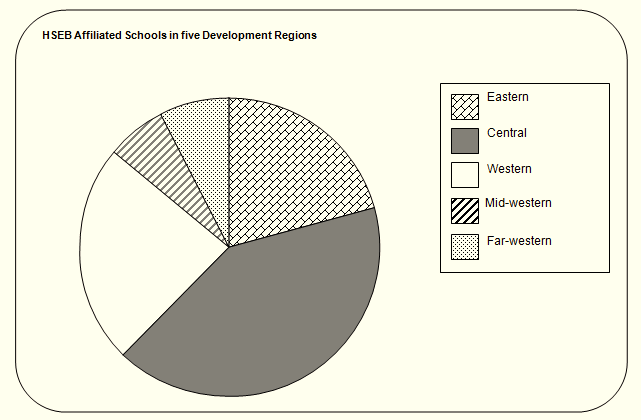# Angular or Pie-Diagram - Economics Notes Grade XI

### Commerce Notes

☰   Related Articles

#### Scarcity And Choice

Concepts of Scarcity And Choice

#### Extra# Angular or Pie-Diagram

Angular or Pie-Diagram:
Pie-diagram is an alternative representation of the data set which can be presented in sub-divided and percentage bars or rectangles. These diagrams are normally used to show the total number of observations of different types of data set inside a circle into various slices according to the magnitudes in terms of angle. Usually, the largest portion of the data in a pie-diagram is shown first at 12 O’clock position on the circle, whereas other observations are shown in clockwise succession in descending order of magnitude. But they can be shown in a logical order as well. The following are the steps to construct a pie-diagram.

1. Find the sum total of all the observations.
2. Convert the data set into corresponding degrees in the circle by using the formula:
Total of observations = 3600 (Since total angle formed by a circle = 3600)3. It should be noted that the sum of the angles converted should be equal to 3600
4. Draw a circle of appropriate size by the help of a compass.
5. Draw a radius at any point on the circle. (Better at 12 O’ clock position).
6. Take the radius drawn as the baseline and draw the successive angles one after another in the circle by the protractor.
7. Different shades or colors can be used to represent various sectors.

Limitations of Pie-diagram:

• It is difficult to calculate the angles with respect to the given data in comparison to the bar diagram.
• It is difficult to construct the pie-diagram in comparison to bar diagram.
• If there are more than 6 characteristics, it is not preferable to construct a pie-diagram.
• In pie-diagram, negative values cannot be presented.

Example:
The HSEB affiliated schools in five development regions are given in the following table. Represent these data by a pie-diagram.

 Development region No. of schools EasternCentralWesternMid-westernFar-western 1653251904960 Total 789

Solution:
Calculation of angles for pie-diagram

 Development region No. of schools Angle (in degrees) EasternCentralWesternMid-westernFar-western 1653251904960 (360/789 165 = 75.8(360/789) 325 = 148.28(360/789) 190 = 86.70(360/789) 49 = 22.36(360/789) 60 = 27.38 Total 789 360Above diagram is the example of Pie-Diagram.

## Popular Subjects

Join with us on social media to see our updates on your feed.